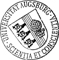## Modeling, simulation, and optimization of surface acoustic wave driven microfluidic biochips

• We will be concerned with the mathematical modeling, numerical simulation, and shape optimization of microfluidic biochips that are used for various biomedical applications. A particular feature is that the fluid flow in the fluidic network on top of the biochips is induced by surface acoustic waves generated by interdigital transducers. We are thus faced with a multiphysics problem that will be modeled by coupling the equations of piezoelectricity with the compressible Navier-Stokes equations. Moreover, the fluid flow exhibits a multiscale character that will be taken care of by a homogenization approach. We will discuss and analyze the mathematical models and deal with their numerical solution by space-time discretizations featuring appropriate finite element approximations with respect to hierarchies of simplicial triangulations of the underlying computational domains. Simulation results will be given for the propagation of the surface acoustic waves on top of the piezoelectricWe will be concerned with the mathematical modeling, numerical simulation, and shape optimization of microfluidic biochips that are used for various biomedical applications. A particular feature is that the fluid flow in the fluidic network on top of the biochips is induced by surface acoustic waves generated by interdigital transducers. We are thus faced with a multiphysics problem that will be modeled by coupling the equations of piezoelectricity with the compressible Navier-Stokes equations. Moreover, the fluid flow exhibits a multiscale character that will be taken care of by a homogenization approach. We will discuss and analyze the mathematical models and deal with their numerical solution by space-time discretizations featuring appropriate finite element approximations with respect to hierarchies of simplicial triangulations of the underlying computational domains. Simulation results will be given for the propagation of the surface acoustic waves on top of the piezoelectric substrate and for the induced fluid flow in the microchannels of the fluidic network. The performance of the operational behavior of the biochips can be significantly improved by shape optimization. In particular, for such purposes we present a multilevel interior point method relying on a predictor-corrector strategy with an adaptive choice of the continuation steplength along the barrier path. As a specific example, we will consider the shape optimization of pressure driven capillary barriers between microchannels and reservoirs.• Dokument_1.pdfAuthor: Harbir AntilGND, Roland GlowinskiGND, Ronald H. W. HoppeGND, Christopher Linsenmann, Tsorng-Whay Pan, Achim WixforthORCiDGND urn:nbn:de:bvb:384-opus4-10642 https://opus.bibliothek.uni-augsburg.de/opus4/1252 Preprints des Instituts für Mathematik der Universität Augsburg (2009-10) Preprint English Universität Augsburg University of Houston, Department of Mathematics 2009/04/28 microfluidic biochips; surface acoustic waves; shape optimization Numerische Strömungssimulation; Biochip; Mathematische Modellierung; Gestaltoptimierung Mathematisch-Naturwissenschaftlich-Technische Fakultät Mathematisch-Naturwissenschaftlich-Technische Fakultät / Institut für Mathematik Mathematisch-Naturwissenschaftlich-Technische Fakultät / Institut für Physik Mathematisch-Naturwissenschaftlich-Technische Fakultät / Institut für Mathematik / Lehrstuhl für Numerische Mathematik Mathematisch-Naturwissenschaftlich-Technische Fakultät / Institut für Physik / Lehrstuhl für Experimentalphysik I 5 Naturwissenschaften und Mathematik / 51 Mathematik / 510 MathematikDeutsches Urheberrecht mit Print on Demand